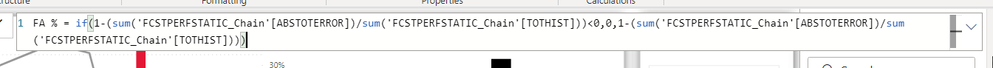cancel
Showing results for
Did you mean:Helper II

## Can you help me understand the formula?

Hello,

I have a DAX calculation that I cannot fully understand. Here is the formula

Does that mean if a result is less than 0 then (1 - (ABSTOERROR / TOTHIST), then it is 0, else the same calculation  (1 - (ABSTOERROR / TOTHIST) ?

Does that mean if we have a negative number we get 0s?1 ACCEPTED SOLUTIONSuper User

Correct, basically, if TOTHIST < ABSTOERROR you will get a number > 1.  When you subtract that from 1 you will get a negative which the formula will return as 0.

2 REPLIES 2Super User

You could rewrite it like this, might make it more clear what is happening.

``````FA % =
VAR _Abs =
SUM ( 'FCSTPERFSTATIC_Chain'[ABSTOTERROR] )
VAR _Hist =
SUM ( 'FCSTPERFSTATIC_Chain'[TOTHIST] )
RETURN
IF ( _Hist < _Abs, 0, 1 - DIVIDE ( _Abs, _Hist ) )``````Super User

Correct, basically, if TOTHIST < ABSTOERROR you will get a number > 1.  When you subtract that from 1 you will get a negative which the formula will return as 0.